# Computing Bundle Segment Diameter

The diameter of bundle segments is based on the diameter of constituent wires and/or wire groups and different formulas are used depending on the number of wires/wire groups routed through the segment.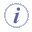## Computing the Diameter of Bundle Segments containing Wire Groups

The diameter of bundle segments containing wire groups is computed in two different ways depending on whether the wire group is routed between two connectors, or between three or more connectors.

Note: The same rules apply when calculating bend radius.

### A. Wire Group between Two Connectors

The bundle segment diameter is based on the wire group.

The formula used depends on the number of wire groups in the bundle segment. In the example below, the bundle segment contains one wire group. The diameter of the bundle segment is the same as that of the wire group.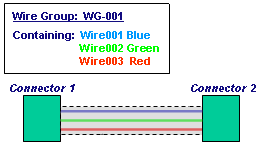Note: If you change the diameter of individual wires, this will not affect the diameter of the wire group and bundle segment.

### B. Wire Group between Three or More Connectors

• If all wires in the group are routed together through the bundle segment (BNS 1 in the illustration below), the bundle segment diameter is based on the wire group.
• If wires in a wire group are routed separately through bundle segments (BNS 2 and 3 in the illustration below), the bundle segment diameter is based on individual wires.

The formula used depends on the number of wire groups and/or wires in the bundle segment.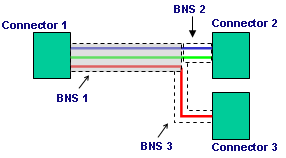## Computing the Diameter of Bundle Segments containing Individual Wires

The bundle segment diameter is computed based on the wires it contains, and bundle segment geometry is updated.
 Before: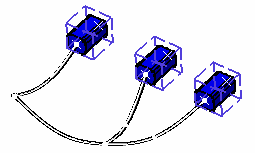After: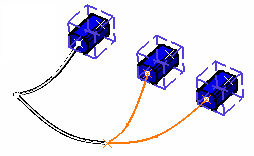The formula used depends on the number of wires in the bundle segment.

### Formula Used

• When the Use equivalent area method to compute bundle segment diameter check box is not selected in the Tools > Options > Equipment & Systems > Electrical Harness Discipline > Electrical Wire Routing > General tab. Number of Wires and/or Wire Groups Bundle Segment Diameter 0 no change 1 wire (or wire group) diameter 2 or 3 2 * Max 4 and above (1 + 2*((N - 1)/3)1/2) * Max where: Max is the diameter of the largest wire (or wire group) N is the number of wires (or wire groups).
• When the Use equivalent area method to compute bundle segment diameter check box is selected in the Tools > Options > Equipment & Systems > Electrical Harness Discipline > Electrical Wire Routing > General tab.

 Number of Wires and/or Wire Groups Bundle Segment Diameter 0 no change 1 wire (or wire group) diameter 2 Sum of wire diameter 3 and above ((1+x)*sum of (wire diameter²))1/2 where 0<= x <= 1 is the percentage added

A typical example:Bundle Segment Constituent Wires and Wire Groups Number of Wires or Wire Groups Bundle Segment Diameter BNS1 All wires in group are routed together 1 Wire group diameter BNS2 Only two wires of the group are routed together 2 2 * diameter of the largest wire BNS3 Only one wire of the group is routed 1 Wire diameter